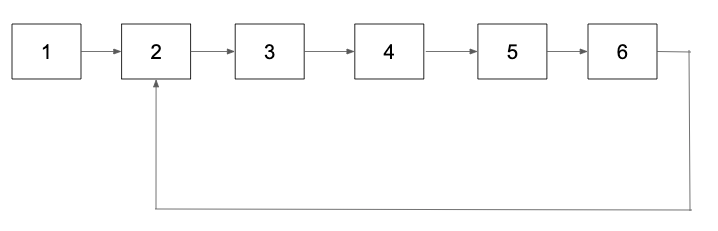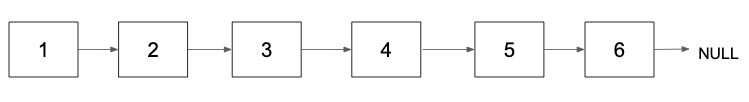Problem title
Difficulty
Avg time to solve

Pair Sum
Easy
15 mins
Maximum XOR With an Element From Array
Hard
50 mins
Word Break-1
Moderate
36 mins
Encode The String
Easy
10 mins
Target Sum
Moderate
--
Nth Fibonacci Number
Easy
--
Detect and Remove Loop
Moderate
10 mins
Level Order Traversal
Easy
15 mins
Last index of element
Easy
--
Remove Duplicates From Sorted List
Easy
--38

# Detect and Remove Loop

Difficulty: MEDIUM
Avg. time to solve
10 min
Success Rate
90%

Problem Statement

#### Input format:

``````The first line of input contains two values: the number of nodes in the linked list and the value of the kth node from which the last node connects to form the loop while the second line of input contains the given linked list.
The value of k should be greater than or equal to 0 and less than equal to n. For, k equal to 0, there is no loop present in the linked list and for k equal to n, the last node is connected to itself to form the cycle.
``````

#### Output Format:

``````The only output line contains the linked list after removing the loop if present.
``````
##### Constraints:
``````1 <= N <= 100000.
1 <= ‘VAL’ <= 1000 .

Time limit: 1 sec
``````

#### Sample Input:

``````6 2
1 2 3 4 5 6
``````

#### Sample Output:

``````1 2 3 4 5 6
``````
##### Explanation:
``````For the given input linked list, the last node is connected to the second node as:
````````````Now, after detecting and removing this loop the linked list will be:
``````Console